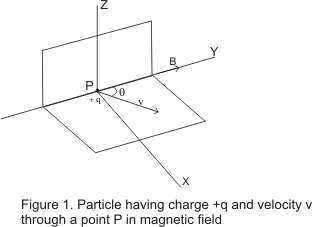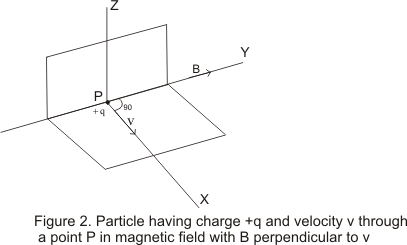# Magnetic Field

## Introduction

• We have already studied about thermal effects of current and now in the present chapter we are studied about magnetic effect of current.
• Earlier it was believe that there is no connection between electric and magnetic force and both of them are completely different.
• But in 1820 Oersted showed that the electric current through a wire deflect the magnetic needle placed near the wire and the direction of deflection of needle is reversed if we reverse the direction of current in the wire.
• So, Oersted's experiments establishes that a magnetic field is associated with current carrying wire.
• Again if we a magnetic needle near a bar magnet it gets deflected and rests in some other direction.
• This needle experiences the torque which turn the needle to a definite direction.
• Thus, the region near the bar magnet or current carrying where magnetic needle experience and suffer deflection is called magnetic field.

## The Magnetic Field

• We all ready know that a stationery charges gets up a electric field E in the space surrounding it and this electric field exerts a force F=q0E on the test charge q0 placed in magnetic field.
• Similarly we can describe the interaction of moving charges that, a moving charge exert a magnetic field in the space surrounding it and this magnetic field exert a force on the moving charge.
• Like electric field, magnetic field is also a vector quantity and is represented by symbol B
• Like electric field force which depend on the magnitude of charge and electric field, magnetic force is proportional to the magnitude of charge and the strength of magnetic field.
• Apart from its dependence on magnitude of charge and magnetic field strength magnetic force also depends on velocity of the particle.
• The magnitude magnetic force increase with increase in speed of charged particle.
• Direction of magnetic force depends on direction of magnetic field B and velocity v of the charged particle.
• The direction of magnetic force is not along the direction of magnetic field but direction of force is always perpendicular to direction of both magnetic field B and velocity v
• Test charge of magnitude q0 is moving with velocity v through a point P in magnetic field B experience a deflecting force F defined by a equation
F=qv X B
• As mentioned earlier this force on charged particle is perpendicular to the plane formed by v and B and its direction is determined right hand thumb rule.• When moving charge is positive the direction of force F is the direction of advancement of right hand screw whose axis is perpendicular to the plane formed by v and B.• Direction of force would be opposite to the direction of advancement of right hand screw for negative charge moving in same direction.
• Magnitude of force on charged particle is
$F=q_0 vBsin \theta$
where θ is the angle between v and B.
• If v and B are at right angle to each other i.e. θ=90 then force acting on the particle would be maximum and is given by
$F_{max}=q_0 vB$ ----(3)
• When θ=180 or θ=0 i.e. v is parallel or anti parallel to B then force acting on the particle would be zero.
• Again from equation 2 if the velocity of the particle in the magnetic field is zero i.e., particle is stationery in magnetic field then it does not experience any force.
• SI unit of strength of magnetic field is Tesla (T). It can be defined as follows
$B=\frac {F}{qvsin \theta}$
for F=1N,q=1C and v=1m/s and θ=90
1T=1NA-1m-1
Thus if a charge of 1C when moving with velocity of 1 m/s along the direction perpendicular to the magnetic field experiences a force of 1N then magnitude of field at that point is equal to 1 Tesla (1T).
• Another SI unit of magnetic field is Weber/m2 Thus
1 Wb-m-2=1T=1NA-1m-1
In CGS system, the magnetic field is expressed in 'gauss'. And 1T= 104 gauss. Dimension formula of magnetic field (B) is [MT-2A-1]